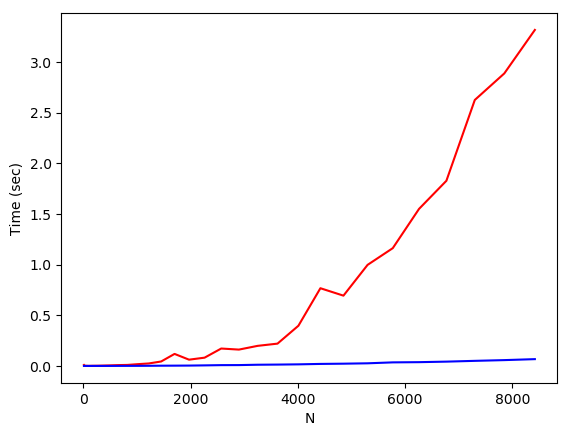## A 5x5 Elimination Matrix, E (l=1, k=3)¶

In :
N = 5;
l = 1;
k = 3;

In :
el=eye(5)[:,l]

Out:
5-element Array{Float64,1}:
1.0
0.0
0.0
0.0
0.0
In :
ek=eye(5)[:,k]

Out:
5-element Array{Float64,1}:
0.0
0.0
1.0
0.0
0.0
In :
el*ek'

Out:
5×5 Array{Float64,2}:
0.0  0.0  1.0  0.0  0.0
0.0  0.0  0.0  0.0  0.0
0.0  0.0  0.0  0.0  0.0
0.0  0.0  0.0  0.0  0.0
0.0  0.0  0.0  0.0  0.0
In :
E=eye(5)+3*el*ek'

Out:
5×5 Array{Float64,2}:
1.0  0.0  3.0  0.0  0.0
0.0  1.0  0.0  0.0  0.0
0.0  0.0  1.0  0.0  0.0
0.0  0.0  0.0  1.0  0.0
0.0  0.0  0.0  0.0  1.0
In :
B=rand(5,5)

Out:
5×5 Array{Float64,2}:
0.64764   0.416888   0.272297  0.829488  0.671648
0.209615  0.0758793  0.97854   0.808906  0.862265
0.291818  0.140414   0.385255  0.823593  0.618398
0.435921  0.248701   0.200266  0.179681  0.204089
0.540153  0.401282   0.632367  0.190665  0.341633
In :
el*ek' * B

Out:
5×5 Array{Float64,2}:
0.291818  0.140414  0.385255  0.823593  0.618398
0.0       0.0       0.0       0.0       0.0
0.0       0.0       0.0       0.0       0.0
0.0       0.0       0.0       0.0       0.0
0.0       0.0       0.0       0.0       0.0     
In :
E * B

Out:
5×5 Array{Float64,2}:
1.52309   0.838129   1.42806   3.30027   2.52684
0.209615  0.0758793  0.97854   0.808906  0.862265
0.291818  0.140414   0.385255  0.823593  0.618398
0.435921  0.248701   0.200266  0.179681  0.204089
0.540153  0.401282   0.632367  0.190665  0.341633

## LU Factorization Implementation: lufact()¶

In :
A=rand(3,3)  ## A random 3x3 matrix

Out:
3×3 Array{Float64,2}:
0.656412  0.88843   0.202747
0.797951  0.222365  0.632138
0.454066  0.277741  0.0591349
In :
?(lufact)  ## help system -- yay!

search: lufact lufact!


Out:
lufact(A [,pivot=Val{true}]) -> F::LU

Compute the LU factorization of A.

In most cases, if A is a subtype S of AbstractMatrix{T} with an element type T supporting +, -, * and /, the return type is LU{T,S{T}}. If pivoting is chosen (default) the element type should also support abs and <.

The individual components of the factorization F can be accessed by indexing:

Component Description
F[:L] L (lower triangular) part of LU
F[:U] U (upper triangular) part of LU
F[:p] (right) permutation Vector
F[:P] (right) permutation Matrix

The relationship between F and A is

F[:L]*F[:U] == A[F[:p], :]

F further supports the following functions:

Supported function LU LU{T,Tridiagonal{T}}
/
\
cond
det
logdet
logabsdet
size
lufact(A::SparseMatrixCSC) -> F::UmfpackLU

Compute the LU factorization of a sparse matrix A.

For sparse A with real or complex element type, the return type of F is UmfpackLU{Tv, Ti}, with Tv = Float64 or Complex128 respectively and Ti is an integer type (Int32 or Int64).

The individual components of the factorization F can be accessed by indexing:

Component Description
F[:L] L (lower triangular) part of LU
F[:U] U (upper triangular) part of LU
F[:p] right permutation Vector
F[:q] left permutation Vector
F[:Rs] Vector of scaling factors
F[:(:)] (L,U,p,q,Rs) components

The relation between F and A is

F[:L]*F[:U] == (F[:Rs] .* A)[F[:p], F[:q]]

F further supports the following functions:

Implementation note

lufact(A::SparseMatrixCSC) uses the UMFPACK library that is part of SuiteSparse. As this library only supports sparse matrices with Float64 or Complex128 elements, lufact converts A into a copy that is of type SparseMatrixCSC{Float64} or SparseMatrixCSC{Complex128} as appropriate.

In [ ]:



## Testing LU¶

In :
F=lufact(A)  ## LU factorization with partial pivoting

Out:
Base.LinAlg.LU{Float64,Array{Float64,2}}([0.797951 0.222365 0.632138; 0.822622 0.705507 -0.317264; 0.56904 0.214323 -0.23258],[2,2,3],0)
In :
F[:L]

Out:
3×3 Array{Float64,2}:
1.0       0.0       0.0
0.822622  1.0       0.0
0.56904   0.214323  1.0
In :
F[:U]

Out:
3×3 Array{Float64,2}:
0.797951  0.222365   0.632138
0.0       0.705507  -0.317264
0.0       0.0       -0.23258 

## Verifying result: A == LU ??¶

In :
F[:L]*F[:U]

Out:
3×3 Array{Float64,2}:
0.797951  0.222365  0.632138
0.656412  0.88843   0.202747
0.454066  0.277741  0.0591349
In :
A  # A != LU --- need to include permutations

Out:
3×3 Array{Float64,2}:
0.656412  0.88843   0.202747
0.797951  0.222365  0.632138
0.454066  0.277741  0.0591349
In :
F[:p]  ## Don't forget to pivot!

Out:
3-element Array{Int64,1}:
2
1
3
In :
A[F[:p], :]   ## Same as L*U

Out:
3×3 Array{Float64,2}:
0.797951  0.222365  0.632138
0.656412  0.88843   0.202747
0.454066  0.277741  0.0591349

## Solving Ax=b¶

In :
N = 4;
A = rand(N,N)

Out:
4×4 Array{Float64,2}:
0.786429   0.429332  0.0624521  0.745315
0.394092   0.425951  0.298966   0.619573
0.0249588  0.321087  0.156631   0.595137
0.88053    0.996113  0.560585   0.121093
In :
b = rand(N)

Out:
4-element Array{Float64,1}:
0.540919
0.724715
0.0625126
0.752182 
In :
LU=lufact(A)

Out:
Base.LinAlg.LU{Float64,Array{Float64,2}}([0.88053 0.996113 0.560585 0.121093; 0.893131 -0.460328 -0.438224 0.637163; 0.0283452 -0.63618 -0.138048 0.997055; 0.447562 0.0431673 -0.485238 1.02168],[4,4,3,4],0)
In :
LU \ b   ## Performs backsubstitution

Out:
4-element Array{Float64,1}:
1.20634
-2.01231
2.94371
0.365381
In :
inv(A)*b

Out:
4-element Array{Float64,1}:
1.20634
-2.01231
2.94371
0.365381
In :
norm(LU \ b - inv(A)*b)/norm(b)

Out:
4.241383723240057e-16
In :
eps()

Out:
2.220446049250313e-16
In :
(norm(LU \ b - inv(A)*b)/norm(b)) / eps()

Out:
1.9101494155519207
In [ ]:



## Timing lufact() and solve ("LU \ b")¶

In :
n = 30
N = 10
timeLU    = zeros(n)
timeSolve = zeros(n)
vecN      = ones(n)
for i = 1:n
A = rand(N,N)
b = rand(N)

# Time LU factorization:
tic()
print(string(N,"x",N,": "))
LU = lufact(A)  ## "!" means compute LU 'in place' - overwrite A
timeLU[i] = toc()
vecN[i] = N

# Time LU backsub solve:
tic()
x = LU \ b
timeSolve[i] = toq()  # quiet toc() -- no printout

# Increase size
N = 10 + 10*round(i^2)
end

10x10: elapsed time: 0.00909094 seconds
20x20: elapsed time: 0.002258116 seconds
50x50: elapsed time: 0.001473188 seconds
100x100: elapsed time: 0.000483221 seconds
170x170: elapsed time: 0.001493419 seconds
260x260: elapsed time: 0.001952619 seconds
370x370: elapsed time: 0.003409656 seconds
500x500: elapsed time: 0.005378073 seconds
650x650: elapsed time: 0.00813408 seconds
820x820: elapsed time: 0.009829342 seconds
1010x1010: elapsed time: 0.01726451 seconds
1220x1220: elapsed time: 0.024421508 seconds
1450x1450: elapsed time: 0.043789634 seconds
1700x1700: elapsed time: 0.118542954 seconds
1970x1970: elapsed time: 0.06170046 seconds
2260x2260: elapsed time: 0.081508947 seconds
2570x2570: elapsed time: 0.17162104 seconds
2900x2900: elapsed time: 0.161139746 seconds
3250x3250: elapsed time: 0.198088675 seconds
3620x3620: elapsed time: 0.219999655 seconds
4010x4010: elapsed time: 0.39649807 seconds
4420x4420: elapsed time: 0.76679111 seconds
4850x4850: elapsed time: 0.693487129 seconds
5300x5300: elapsed time: 0.997214341 seconds
5770x5770: elapsed time: 1.162193135 seconds
6260x6260: elapsed time: 1.549786969 seconds
6770x6770: elapsed time: 1.827127481 seconds
7300x7300: elapsed time: 2.624738668 seconds
7850x7850: elapsed time: 2.886459943 seconds
8420x8420: elapsed time: 3.315852673 seconds

In [ ]:



## Plotting Time vs N¶

In :
using PyPlot

In :
plot(vecN, timeLU, "r-")
plot(vecN, timeSolve, "b-")
xlabel("N")
ylabel("Time (sec)")Out:
PyObject <matplotlib.text.Text object at 0x7f09a6567390>
In [ ]: